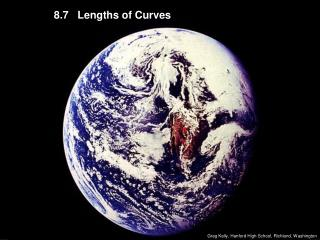DownloadDownload Presentation8.7 Lengths of Curves

# 8.7 Lengths of Curves

Download Presentation## 8.7 Lengths of Curves

- - - - - - - - - - - - - - - - - - - - - - - - - - - E N D - - - - - - - - - - - - - - - - - - - - - - - - - - -
##### Presentation Transcript

1. 8.7 Lengths of Curves Greg Kelly, Hanford High School, Richland, Washington

2. Length of Curve (Cartesian) Lengths of Curves: If we want to approximate the length of a curve, over a short distance we could measure a straight line. By the pythagorean theorem: We need to get dx out from under the radical.

3. Yucko!Now what? This doesn’t fit any formula, and we started with a pretty simple example! Example: The TI-Nspire CAS gets:

4. If we check the length of a straight line: Example: The curve should be a little longer than the straight line, so our answer seems reasonable.

5. Example: You may want to let the TI-Nspire CAS find the derivative too: 1st define y = -x2 + 9 in one of 3 ways. Pick your favorite. i) Define y = -x2 + 9 (b, 1:Actions, 1:Define) or ii) y:= - x2 + 9 (: is middle of right column of keys; = is on the top left) or iii) –x2 + 9 /hy /r Then press choose 0e3e /q1+ /r choose XeYeqeeX /·

6. Example: Before you do the next problem on your Nspire you should go to Tools (/c), 4:Insert, 1:Problem or to clear the variable in a-z you could press b, 1:Actions, 4:Clear a-z

7. If you have an equation that is easier to solve for x than for y, the length of the curve can be found the same way. Notice that x and y are reversed. Try this on your Nspire